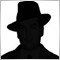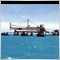• 概要
• レビュー (4)
• コメント

# K Channel

K_Channel is a technical indicator defining the current Forex market status - trend or flat.

The indicator works on any timeframe. H1 and higher timeframes are recommended though to minimize false signals.

The indicator is displayed as lines above and below EMA. Average True Range (ATR) is used as bands' width.

Therefore, the channel is based on volatility. This version allows you to change all the parameters of the main Moving Average.

Unlike Bollinger Bands that applies the standard deviation value, К_Channel uses maximum and minimum prices within a period.

K_Channel indicator is calculated by the following formula:

Upper Level = EMA13 (Typical Price) + EMA13 (Trading Range)

Lower Level = EMA13 (Typical Price) - EMA13 (Trading Range),

Trading Range = max(high(t), low(t))- min(high(t), low(t)), where

high(t) - highest price of the current period,

low(t) - lowest price of the current period.

### Parameters

• period - number of bars used for the indicator calculations;
• method - select from the four Moving Averages;
• apply to - select from the seven applied price types.17216
Nikolay Gaylis 2019.01.14 06:47

ユーザーはレーティングに対して何もコメントを残しませんでした12014
2017.12.14 20:45

ユーザーはレーティングに対して何もコメントを残しませんでした5507
2017.11.09 03:06

ユーザーはレーティングに対して何もコメントを残しませんでした6084
2016.12.21 07:25

ユーザーはレーティングに対して何もコメントを残しませんでした